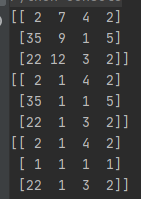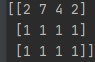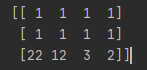• 即把程序的array_a换成list_a，则会报错： d = list_a[list_b] TypeError: list indices must be integers or slices, not list e = list_a[array_b] TypeError: only integer scalar arrays can be converted to ...
首先上一段程序：
import numpy as np
list_a = list(range(10))
print("list_a: {}".format(list_a))
array_a = np.array(list_a)
print("array_a: {}".format(array_a))
list_b = [True,False,True,True,False,False,True,False,True,False]
print("list_b: {}".format(list_b))
array_b = np.array(list_b)
print("array_b: {}".format(array_b))
下面是这段程序的输出：
list_a: [0, 1, 2, 3, 4, 5, 6, 7, 8, 9]
array_a: [0 1 2 3 4 5 6 7 8 9]
list_b: [True, False, True, True, False, False, True, False, True, False]
array_b: [ True False True True False False True False True False]
mask: [False False False False False False False False False False]
从输出可以看出list和array的不同之处是list是以逗号作为分隔符，而array是以空格作为分隔符。还有numpy中的zeros生成的是array。其实，list和array还有很多的不同之处，例如：
list是Python中的普通列表对象，支持append和attend操作，没有shape属性；array是numpy数据库中的对象，不支持append和attend操作，具有shape属性。
一个list中可以存放不同类型的数据，如int、float、str或者布尔型；而array中只能存放相同类型的数据。
list不支持乘法操作；array支持乘法操作。
而对于赋值操作，操作对象只能是array,而索引可以是array也可以是list：
d = array_a[list_b]
print("array_a[list_b]: {}".format(d))
e = array_a[array_b]
print("array_a[array_b]: {}".format(e))
输出如下：
array_a[list_b]: [0 2 3 6 8]
array_a[array_b]: [0 2 3 6 8]
mask: [ True False True True False False True False True False]
此番操作可以将array_a中对应b数组或列表True位上的元素组成一个新的数组。
如果对列表进行如此赋值操作，即把程序中的array_a换成list_a，则会报错：
d = list_a[list_b]
TypeError: list indices must be integers or slices, not list
e = list_a[array_b]
TypeError: only integer scalar arrays can be converted to a scalar index
以上这篇python之array赋值技巧分享就是小编分享给大家的全部内容了，希望能给大家一个参考，也希望大家多多支持我们。
本文标题: python之array赋值技巧分享
本文地址: http://www.cppcns.com/jiaoben/python/289341.html
展开全文• 您可以使用b=numpy.copy(a)然后是b[numpy.isnan(b)]=1In : a[:] = numpy.NaNIn : b=numpy.copy(a)In : b[numpy.isnan(b)]=1In : aOut:array([[ nan, nan, nan],[ nan, nan, nan],[ nan, nan, ...
您可以使用
b=numpy.copy(a)然后是b[numpy.isnan(b)]=1
In : a[:] = numpy.NaN
In : b=numpy.copy(a)
In : b[numpy.isnan(b)]=1
In : a
Out:
array([[ nan, nan, nan],
[ nan, nan, nan],
[ nan, nan, nan]])
In : b
Out:
array([[ 1., 1., 1.],
[ 1., 1., 1.],
[ 1., 1., 1.]])使用b =a[:]将不适用于您的示例，如果更改任一数组，值将更改。
In : import numpy
In : a = numpy.empty((3,3,))
In : a[:] = numpy.NaN
In : a
Out:
array([[ nan, nan, nan],
[ nan, nan, nan],
[ nan, nan, nan]])
In : b=a[:]
In : b
Out:
array([[ nan, nan, nan],
[ nan, nan, nan],
[ nan, nan, nan]])
In : b[numpy.isnan(b)]=1
In : a
Out:
array([[ 1., 1., 1.],
[ 1., 1., 1.],
[ 1., 1., 1.]])
In : b
Out:
array([[ 1., 1., 1.],
[ 1., 1., 1.],
[ 1., 1., 1.]])
In : a[:] = numpy.NaN
In : a
Out:
array([[ nan, nan, nan],
[ nan, nan, nan],
[ nan, nan, nan]])
In : b
Out:
array([[ nan, nan, nan],
[ nan, nan, nan],
[ nan, nan, nan]])
展开全文• 定义矩阵A后，给变量t赋值为A矩阵的第二行向量，但是当改变原本矩阵第二行向量，变量t也发生改变。请问如果修改代码可以让t的值不被覆盖呢？ 谢谢！！！  A = np.array([1,1,3,2,3,0]).reshape(2,3) ...
• 我在Python2.6.5有一个关于numpy的奇怪问题。我分配了一个numpy数组，然后将一个新变量等效到它。当我对新数组执行任何操作时，原始数组的值也会更改。为什么会这样？请参阅下面的示例。请启发我，因为我对Python...
我在Python2.6.5中有一个关于numpy的奇怪问题。我分配了一个numpy数组，然后将一个新变量等效到它。当我对新数组执行任何操作时，原始数组的值也会更改。为什么会这样？请参阅下面的示例。请启发我，因为我对Python和一般编程还比较陌生。
-苏扬
1
2
3
4
5
6
7
8
9
10
11
12
13
14
15
16
17
18
19
20
21>>> import numpy as np
>>> a = np.array([[1,2],[3,4]])
>>> b = a
>>> b
array([[1, 2],
[3, 4]])
>>> c = a
>>> c
array([[1, 2],
[3, 4]])
>>> c[:,1] = c[:,1] + 5
>>> c
array([[1, 7],
[3, 9]])
>>> b
array([[1, 7],
[3, 9]])
>>> a
array([[1, 7],
[3, 9]])
这实际上根本不是问题，而是数组(和其他对象)在Python中的工作方式。
请这样想：您在代码示例中创建的数组是一个位于内存中某个位置的对象。但是你不能在你的程序中通过告诉python在内存中的哪个位置去寻找它来使用它；你必须给它一个名字。当你写作时
1a = np.array([[1,2],[3,4]])
您正在创建数组并创建引用该数组的名称a。从那时起，python就知道a指的是"内存地址0x123674283"(或其他)。python运行时中有一个包含所有这些信息的内部表(如果我记得正确的话，称为"符号表")，所以在上面一行python代码运行之后，这个表将包含
1
2
3...,
'a' : 0x123674283,
...
当您将一个变量的值赋给另一个变量时，例如
1b = a
python不会复制整个数组，因为如果它是一个大数组，则需要很长时间。相反，它会转到符号表，并将a的内存地址复制到b表中的新行。所以你最终
1
2
3
4
5...,
'a' : 0x123674283,
...,
'b' : 0x123674283,
...
你看，a和b实际上指的是内存中相同的位置，即相同的对象。您对其中一个所做的任何更改都将反映在另一个中，因为它们只是同一事物的两个名称。
如果要实际复制数组，则必须调用一个方法来显式执行该操作。numpy数组有一个copy方法，您可以仅用于此目的。所以如果你写
1b = a.copy()
然后，python将首先实际复制数组——也就是说，它留出了一个新的内存区域，比如在地址0x123904381处，然后转到内存地址0x123674283，并将数组的所有值从内存的后一部分复制到前一部分。所以你在记忆中的两个不同的地方有相同的内容。
1
2
3
4
5...,
'a' : 0x123674283,
...,
'b' : 0x123904381,
...
现在，当您更改b的一个元素时，该更改不会出现在a中，因为a和b不再指计算机内存的同一部分。由于阵列数据有两个独立的副本，因此可以更改其中一个而不影响另一个。
非常感谢您的精彩描述。不用说，这解决了问题，但我真的很感谢你花时间解释。你启发了我！
"python不会复制整个数组，因为如果它是一个大数组，则需要很长的时间。"—我想说，它所花的时间更少，如果不以这种方式实现，则需要引入一些类似于单独指针类型的东西，这些东西会使事情变得复杂。
简言之，变量赋值创建对现有对象的新引用。
1
2A = object # A points to object in memory
B = A # B points to the same object
展开全文• a[:,]=c 给矩阵的第2列赋值为1，更新a 然后a[,:]=d 给更新过的矩阵a的第2行赋值为1。 importnumpy as np a=np.array([[ 2, 7, 4, 2], [35, 9, 1, 5], [22, 12, 3, 2]])print(a) f=np.array([[ 1, 1, 1, 1], [1...
importnumpy as np
a=np.array([[ 2, 7, 4, 2],
[35, 9, 1, 5],
[22, 12, 3, 2]])print(a)#b=a[np.lexsort(a[:,::-1].T)]#print(b)
c=np.array([[1,1,1]]).T
a[:,]=cprint(a)
d=np.array([1,1,1,1])
a[,:]=dprint(a)
a[:,]=c 给矩阵的第2列赋值为1，更新a
然后a[,:]=d 给更新过的矩阵a的第2行赋值为1。importnumpy as np
a=np.array([[ 2, 7, 4, 2],
[35, 9, 1, 5],
[22, 12, 3, 2]])print(a)
f=np.array([[ 1, 1, 1, 1],
[1, 1, 1, 1],])
a[[1,2],:]=fprint(a)
给矩阵a的第2行和第3行赋值全为1importnumpy as np
a=np.array([[ 2, 7, 4, 2],
[35, 9, 1, 5],
[22, 12, 3, 2]])print(a)
g=np.array([[ 1, 1, 1, 1],
[1, 1, 1, 1]])
a[0:2,:]=fprint(a)
给矩阵a的第一行开始，算上第一行向后数2-0=2行，也就是第一行到第二行全部赋值为1`
展开全文• 所以在numpy选择整行的正确语法是M[row_number, :]：部分只是以速记的方式选择整行。还有一种可能是使用m:，让它从某个索引转到末尾，其中m是一些已知的索引。如果您想在已知索引之间切换，那么我们将使用M[row_...
• 主要介绍了python关于矩阵重复赋值覆盖问题的解决方法,涉及Python深拷贝与浅拷贝相关操作与使用技巧,需要的朋友可以参考下
• 我刚刚学习python不久，有一些小小的...但是在给矩阵赋值时，就要使用 例如把一个矩阵里的值赋值给另一个矩阵： 以上内容是用np.mat创建矩阵时的操作，个人理解不深，仅供参考 np.array创建矩阵时应该有所不同。 ...
• 本文实例讲述了Python表示矩阵的方法。分享给大家供大家参考，具体如下：在c语言，表示个“整型3行4列”的矩阵，可以这样声明：int a;在python中一不能声明变量int，二不能列出维数。可以利用列表夹带列表...
• 2Youki使用索引快速为数组或矩阵赋值的技巧 2.1 构造索引数组获取对角线元素构成的数组 今天在学习CV的时候，需要对矩阵的对角线元素进行赋值，那么首先需要解决的是需要获得矩阵的对角线元素， 用循环是可以的，...
• 在 tensorflow tf.identity 用于返回一个与输入 tensor 值相同的 tensor。 下面程序要做的是，5次循环，每次循环给x加1，赋值给y，然后打印出来 x = tf.Variable(0.0) #返回一个op，表示给变量x加1的操作 x_...tensorflow
• 话不多说，直接上题 @lke 说：你好，我运行了这段代码，我想得到的是一个三维矩阵，可是输出的结果却是矩阵里面还包含了一个数组。 我的思路是将t1与t2连接起来，然后循环赋值给input数组，请问有什么问题吗？ 输出...
• python 循环数据赋值实例python在数值赋值的时候可以采用数值内循环赋值，很方便如下a = [x for x in range(10)]这样a = [0,1,2,3,4,5,6,7,8,9]这里循环得到的是x，x是每次循环的值，这里就是0123456789如果需要用到...
• 鉴于最近复习线性代数计算量较大，且1800答案常常忽略一些逆阵、行列式的计算答案，故用python写出矩阵的简单计算程序，便于检查出错的步骤。 1、行列式 可自行更改阶数from numpy import * # 求行列式 ,建议：取...
• 展开全部在Python中，一2113个像这样的多维表格可以通过“5261序列的序列”4102实现。一个表格是行的序1653列。每一行又是独立单元格的序列。这类似于我们使用的数学记号，在数学里我们用Ai,j，而在Python里我们使用...
• 原标题：问答 | python 矩阵输出问题这里是 AI 研习社，我们的问答版块已经正式推出了！欢迎大家来多多交流~http://ai.yanxishe.com/page/question（文末有福利哦）社长为你推荐来自 AI 研习社问答社区的精华问答。...
• 给定一个矩阵A B=A C=A 为什么我用循环更改了C的值，B和A也一起变了，有朋友知道为什么么。。。。
• 前两天学了学卡尔曼滤波的原理，想应用到实例，原理上写出了各种矩阵。但是赋完值矩阵相乘的时候，总是出现错误。考虑是矩阵维度的问题。 输出了一下各矩阵的维度： 调整了一下 就ok。 ...
• python 下三角矩阵A matrix can be seen in different ways and one of them is the upper triangular matrix part. Some problems in linear algebra are concerned with the upper triangular part of the matrix....numpy 机器学习 深度学习 人工智能
• Python中的数据类型 Python的‘一切皆对象’的具体说明 所以：Python如何对一个对象进行复制？[浅复制、深复制] 关于Python的‘一切皆对象’     ‘一切皆对象’说的是python内的变量，不管数据...深复制 对象 数据类型
• 广告关闭腾讯云11.11云上盛惠...python的numpy创造矩阵from numpy import matimport numpy asnpdata1=mat(zeros((3,3))); #创建一个3*3的零矩阵矩阵这里zeros函数的参数是一个tuple类型(3,3)data2=mat(ones((2,4))...
• ## numpy矩阵赋值问题

万次阅读 2018-12-17 18:09:06
矩阵赋值时需要注意的问题： import numpy as np a = np.array([[1,2,3,4]]) b = a b[0,1] = 0 输出为 import numpy as np a = np.array([[1,2,3,4]]) b = np.copy(a) b[0,1] = 0 输出为 区别很明显了 ...
• 本文实例讲述了Python操作多维数组输出和矩阵运算。分享给大家供大家参考，具体如下：在许多编程语言（Java，COBOL，BASIC），多维数组或者矩阵是（限定各维度的大小）预先定义好的。而在Python中，其实现更简单...
• 先求出扩充矩阵的维度，再按照每一行每一列遍历，根据元素的索引规律依次赋值，最终实现新的扩充矩阵。这个思路实现如下： import numpy as np def PadMat(Ndim, Mat): Brow = Bcol = 2*Ndim-1 B = np.zeros(......

# python中矩阵赋值python 订阅